• 两种方法： 传统的递归快速排序 采用非递归堆栈模拟
• java 快速排序非递归正确版
static public void main(String[] args)
{
int array[]={3,0,12,8,6,1,7,9,2,4};
quickSort(array,0,array.length-1);
//nonRec_quickSort(array,0,array.length-1);
printArray(array);
}

static private void quickSort(int array[],int start,int end){
Stack<Integer> stack=new Stack<>();
stack.push(start);
stack.push(end);

int key;
while(!stack.isEmpty()){

int _end=stack.pop();
int _start=stack.pop();

key=array[_start];

int l=_start;
int h=_end;

while(l<h){

while(array[h]>=key&&l<h)
h--;

while(array[l]<=key&&l<h)
l++;

if(l<h) {
int temp1 = array[h];
array[h] = array[l];
array[l] = temp1;
}

}

if(key>array[l])
{

int temp=array[_start];
array[_start]=array[l];
array[l]=temp;
}

if(_start<l-1)
{
stack.push(_start);
stack.push(l-1);
}

if(_end>l+1)
{
stack.push(l+1);
stack.push(_end);
}

}
}
展开全文• import java.net.PasswordAuthentication; import java.util.Arrays; import java.util.HashMap; import java.util.Map; import java.util.Stack; public class quickSort2 { public static void q...
package link;

import java.util.Arrays;
import java.util.HashMap;
import java.util.Map;
import java.util.Stack;

public class quickSort2 {
public static void quickSort(int[] arr,int startIndex,int endIndex) {
//用一个集合栈来代替递归函数栈
Stack<Map<String,Integer>> quickSortStack=new Stack<Map<String,Integer>>();
//整个数列的起止下标，以哈希的形式入栈
Map rootParam=new HashMap();
rootParam.put("startIndex", startIndex);
rootParam.put("endIndex", endIndex);
quickSortStack.push(rootParam);
//循环结束条件：栈为空
while(!quickSortStack.isEmpty()) {
//栈顶元素出栈，得到起止下标
Map<String,Integer> param=quickSortStack.pop();
//得到基准元素的位置
int pivotIndex=partition(arr,param.get("startIndex"),param.get("endIndex"));
//根据基准元素分成两个部分，把每一部分的起止下标入栈
if(param.get("startIndex")<pivotIndex-1) {
Map<String,Integer> leftParam=new HashMap<String,Integer>();
leftParam.put("startIndex", param.get("startIndex"));
leftParam.put("endIndex", pivotIndex-1);
quickSortStack.push(leftParam);
}
if(pivotIndex+1<param.get("endIndex")) {
Map<String, Integer> rightParam=new HashMap<String ,Integer>();
rightParam.put("startIndex", pivotIndex+1);
rightParam.put("endIndex", param.get("endIndex"));
quickSortStack.push(rightParam);
}
}

}

private static int partition(int[] arr,int startIndex,int endIndex) {
//取第一个位置（也可以选择随机位置）的元素作为基准元素
int pivot=arr[startIndex];
int mark=startIndex;
for (int i = startIndex+1; i <=endIndex; i++) {
if(arr[i]<pivot) {
mark++;
int p=arr[mark];
arr[mark]=arr[i];
arr[i]=p;
}
}
arr[startIndex]=arr[mark];
arr[mark]=pivot;
return mark;
}
public static void main(String[] args) {
int[] arr=new int[] {4,7,6,5,3,2,8,1};
quickSort(arr, 0, arr.length-1);
System.out.println(Arrays.toString(arr));
}

}

展开全文• 问题当数据量较大时（数组长度1000000），快速排序递归算法栈溢出，且实际运行时间也比其他算法长，我猜测是由于大量的函数调用。有人能分析一下吗？如何改进？递归private void quick_sort_recursive(int head, int...
问题
当数据量较大时（数组长度1000000），快速排序递归算法栈溢出，且实际运行时间也比其他算法长，我猜测是由于大量的函数调用。有人能分析一下吗？如何改进？
递归
private void quick_sort_recursive(int head, int rear) {
int j = rear;
while (i != j) {
while (i < j && array[j] >= tmp) j--;
while (i < j && array[i] <= tmp) i++;

if (i < j) {
array[i] = array[i] + array[j];
array[j] = array[i] - array[j];
array[i] = array[i] - array[j];
}
}
array[i] = tmp;

quick_sort_recursive(i + 1, rear);

}

public void quickSortRecursive() {
quick_sort_recursive(0, len - 1);
}

非递归
private void quick_sort_not_recursive(int head, int rear) {
Stack<Integer> stack = new Stack<Integer>();
stack.push(rear);
int i, j;
while (!stack.empty()) {
rear = stack.pop();
j = rear;

while (i != j) {
while (i < j && array[j] >= key) j--;
while (i < j && array[i] <= key) i++;
if (i < j) {
array[i] = array[i] + array[j];
array[j] = array[i] - array[j];
array[i] = array[i] - array[j];
}

}
array[i] = key;

if (i - 1 > head) {
stack.push(i-1);
}
if (i + 1 < rear) {
stack.push(i+1);
stack.push(rear);
}

}
}

public void quickSortNotRecursive(){
quick_sort_not_recursive(0,len-1);
}


展开全文• 快速排序Java版） 听名字就很diao，直接上干货。（杠精别打我，支点的英文是pivot，我全拼错了） 快排一定要思路清晰，没事多写几遍，要不容易忘。 对于一个数组对它进行排序，快排的思想就是交换交换再交换，我们...
快速排序（Java版）
听名字就很diao，直接上干货。
快排一定要思路清晰，没事多写几遍，要不容易忘。这个程序是从小到大排序！！！
对于一个数组对它进行排序，快排的思想就是交换交换再交换，我们先选择一个支点 int pivot，这个支点的作用是，我们要将一个什么都不是的数组，经过第一遍快排，以这个支点就是数组中的某一个元素（一般以数组的第一个元素选择为支点）为分界线，这个支点的左边都比它小，右边都比它大，于是我们的数组经过第一遍快排，分为了三部分，比支点小的数----支点----比支点大的数。实现的方法就是元素之间进行交换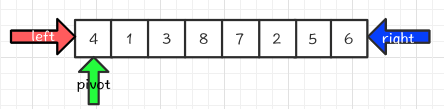这是一个没排序的数组，我们选取4为支点（一般都选取数组的第一个元素），必不可少的还有左右边界的元素的下标，因为交换肯定要知道至少两个元素的下标，我们先把4和right指向的元素进行比较，4<6，我们从小到大排序，所以不用交换，因为大的数肯定在右边。所以right–，向下走看看有没有比4小的。得到下面的图：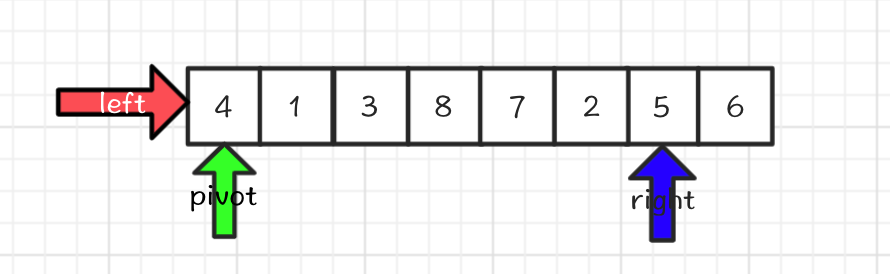此时我们发现4<5，所以right–。得到下图：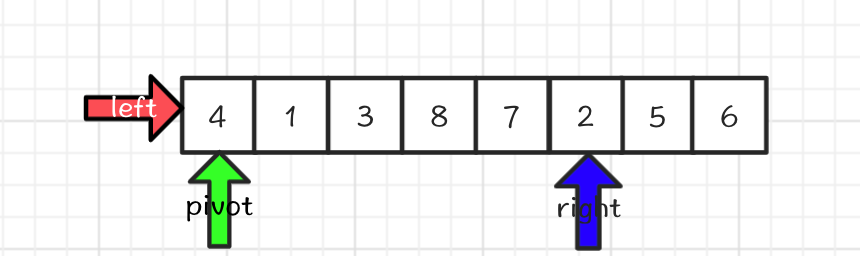到这一步我们发现4>2，按照快排的规则，我们交换两个数，因为小的数一定要在支点的左边。于是得到下图：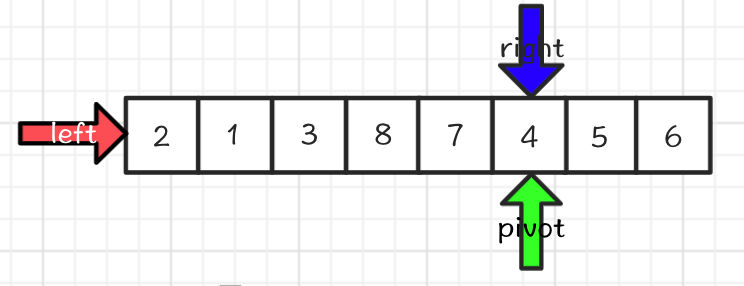我们交换结束后，此时注意，轮到left指针了，left指向2，2<4，满足小的数在支点的左边，所以left++，去寻找比支点大的数进行交换，得到下图：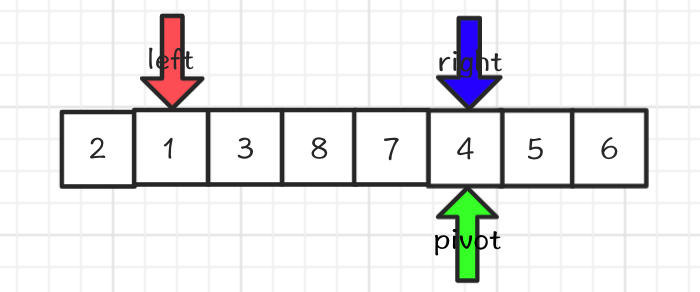1<4，满足条件，所以left++寻找比支点大的数，得到下图：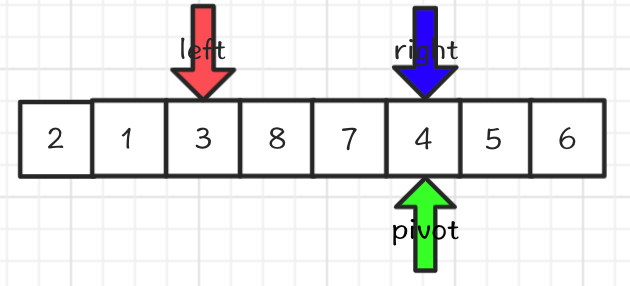3<4，满足条件，所以left++寻找比支点大的数，得到下图：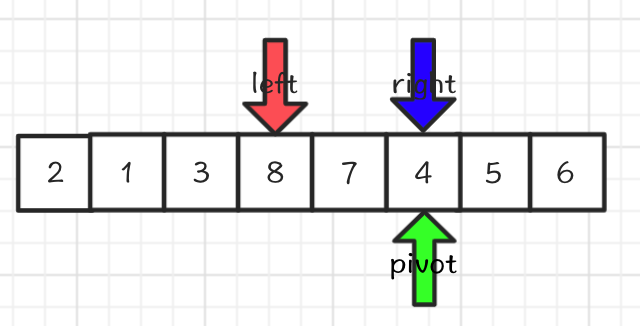到这一步，8>4，所以8应该去支点的右边，得到下图：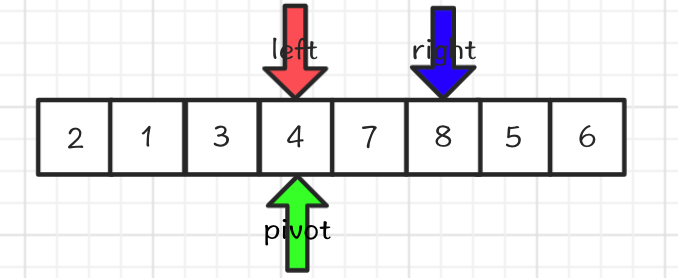此时，又该right指针移动，8>4，满足条件，所以right–，得到下图：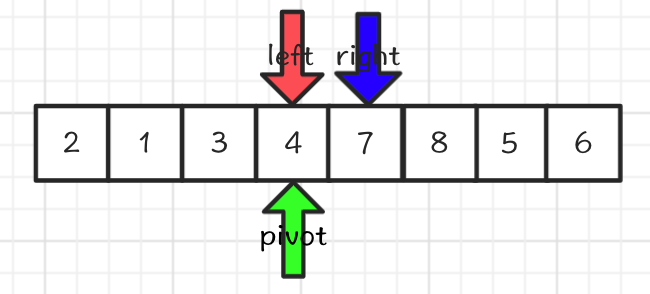此时7>4也满足条件，所以right–，得到下图：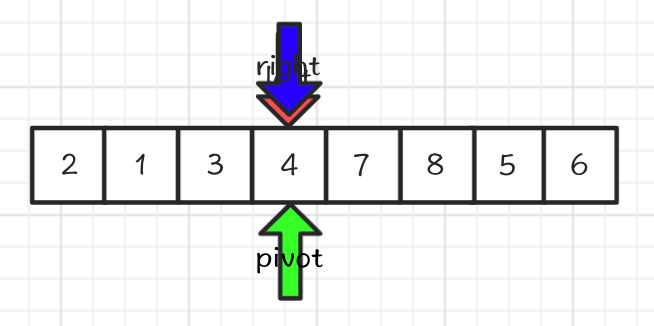这就是循环终止的条件，left==right，此时我们可以发现，在支点4的左边，全部比4小，右边比4大，所以这就是快排的一趟，主要是把数组分开，那么下一步该怎么做呢，其实可以用递归，这时候，4左边的数可以构成一个数组，右边也可以构成一个数组，对这两边的内容再进行上面的步骤，最后呈现出来的，就是从小到大的完整数组，如下图：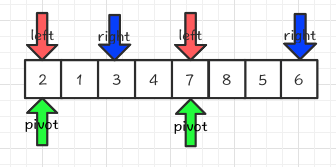到这里就不继续向下说了，就是把上面的流程又走一遍。
总结一下就是，选取第一个元素作为支点，从right指向的值与支点比较，如果支点的值小于right指向的值，right–，直到遇到支点的值大于right指向的值，那么交换right的值和privot的值，一旦一交换，立刻开始比较left与支点的值，left比支点小，left++，否则交换，然后再用right的值与支点比较…一旦交换元素，就要切换比较的对象，right变left，left变right…
直接上代码
递归
/**
*
* @author Coding6
*	快速排序递归
*/
public class QuickSortDemo {
public void sort(int []arr, int left, int right) {
int privot;
if(left<right) {
privot = QuickSort(arr, left, right);
sort(arr, left, privot-1);
sort(arr, privot+1, right);
}
}
public int QuickSort(int []arr, int left, int right) {
int pivot = arr[left];
while(left < right){
while(left < right && pivot >= arr[right]){
right--;
}
arr[left] = arr[right];
while(left < right && pivot <= arr[left]){
left++;
}
arr[right] = arr[left];
}
arr[left] = pivot;
return left;
}
public static void main(String[] args) {
QuickSortDemo q = new QuickSortDemo();
int arr[] = {5,3,6,7,2,4,1,32,67,10,45};
q.sort(arr, 0, arr.length-1);
for (int i = 0; i < arr.length; i++) {
System.out.print(arr[i]+" ");
}
}
}

QuickSort函数，表示的就是一趟的快排
非递归
用到栈
import java.util.Stack;

/**
* @author Coding6
* 快速排序非递归
*/
public class QuickSortStack {
public void sort(int []arr, int left, int right) {
int privot, top, last;
Stack<Integer> s = new Stack<Integer>();
last=0;
top=0;
privot=QuickSort(arr, left, right);
if(privot>left+1) {
s.push(left);
s.push(privot-1);

}
if(privot<right-1) {
s.push(privot+1);
s.push(right);
}
while(!s.empty()) {
top = s.pop();
last = s.pop();
privot = QuickSort(arr, last, top);
if(privot>last+1) {
s.push(last);
s.push(privot-1);
}
if(privot<top-1) {
//System.out.println(top);
s.push(privot+1);
s.push(top);
}
}
}
public int QuickSort(int []arr, int left, int right) {
int privot = left;
while(left<right) {
while((arr[privot]<=arr[right])&left<right) {
right--;
}
int temp = arr[privot];
arr[privot] = arr[right];
arr[right] = temp;
privot = right;

while((arr[privot]>=arr[left])&left<right) {
left++;
}
temp = arr[privot];
arr[privot] = arr[left];
arr[left]  = temp;
privot  = left;
}
return privot;
}
public static void main(String[] args) {
QuickSortStack q = new QuickSortStack();
int arr[] = {5,3,6,7,2,4,1,32,67,10,45};
q.sort(arr, 0, arr.length-1);
for (int i = 0; i < arr.length; i++) {
System.out.print(arr[i]+" ");
}
}
}

展开全文• 快速排序 的原理及其java实现（递归与非递归递归
• * 快速排序 * */ public class QuickSort{ /** * 递归一 * */ public static void sort1(int[] arr, int start, int end) { if(start ){ //初始先将第一个值 int pvoit = arr[start]; int ...
• 快速排序使用“分而治之”的思想，在待排序的元素中选取某一元素作为基准值，通过一趟快速排序将待排序序列分割成两部分，基准值左边部分的所有元素均小于基准值，右边部分的所有元素均大于基准值，之后分别对这两...
• 快速排序非递归实现及递归实现性能比较 快排是常用算法，本文不再赘述快排原理。本文主要研究快速排序非递归实现及递归实现在排序900万个int整数时的性能差异。 一 代码 package common.algorithm; import ...
• 快速排序由于排序效率在同为O(N*logN)的几种排序方法中效率较高，因此经常被采用，再加上快速排序思想----分治法也确实实用，因此很多软件公司的笔试面试，包括像腾讯，微软等知名IT公司都喜欢考这个，还有大大小的...算法
• 基础总结一下，快速排序的步骤： 1、找到一个key值（就是数组第一个值），先从右到左找，找到一个比它小的值，记录下标。 2、然后从左往右找，找到一个比它大的值，记录下标。 3、交换找到的两个数字。 4、继续...算法
• 快速排序的算法思想、动态演示 、C++、python实现，请参考快速排序详解 快速排序也是一种选择排序。 快速排序的思想：将序列分为两部分，左边一部分比它小，右边一部分比它大。然后递归。这里的它，指的是基数。 ...
• Java非递归方式实现快速排序 1 2 3 4 5 6 7 8 9 10 11 12 13 14 15 16 17 18 19 20 21 22 23 24 25 26 27 28 29 30 31 32 33 34 35 36 37 38 39 40 41 42 43 44 45 46 47 48 49 50 51 52 53 54 55 ...
• 非递归实现时借助栈， package sort; import java.util.Stack; public class Sort { /*  * 非递归版本  */  public void quick2(int[] array){  if (array == null || array.length == 1) return...
• 快速排序的思想： 1.从数列中选取一个元素称为“基准值”（最基本的快速排序选取待排序数组的第一个数字作为基准值）。 2.对数列进行排序，将比基准值小的数放在基准前面，比基准值大的数放在基准后面（相同的数可以...
• 算法思想： ​ 在数组中选出一个元素做枢轴，把所有比它小的元素移...排序过程： 递归版： import java.util.Arrays; public class QuickSort { public static void main(String[] args) { int a[] = new in...数据结构 算法
• 挖坑法递归 void quicksort(int s[],int left,int right){ if(left<right){ int temp,i=left,j=right; temp=s[right]; while(i<j){ //...
• 快速排序是大多数公司面试人员的必考题，今天将自己参考别人的思想所写的两种快速排序的方式； 快速排序使用场景：无序列表，时间复杂度为O(log2N)； 快速排序的思想： 3.1、在一个数组中选取一个基准数，根据这...
• ## 快速排序非递归java

千次阅读 2017-06-30 13:01:59
private static void quickSort(int[] a, int start, int end) { LinkedList<Integer> stack = new LinkedList(); // 用栈模拟 if (start ) { stack.push(end); s
• 文章目录1. 基本思想2. 代码实现2.1 递归实现2.2 优化—非递归实现3. 性能分析 1. 基本思想 在数列排序中，如果只有一个数，那么它本身就是有序的；如果只有两个数，那么一次... 快速排序多种递归、非递归实现及性能
• 快速排序算法 工作之前一直不懂快速排序算法，今天看了下快速排序算法，跟大家分享下，如果有不妥之处还请建议。 快速排序是对冒泡排序的一种改进，由C.R.A.Hoare于1962年提出，它采用了一种分治的策略，通常称...算法
• import java.util.Arrays;... * 采用非递归的方法，首先要想到栈的使用，通过阅读递归调用部分的代码，思考如何用栈来代替。 * 递归调用的核心代码是 temp = partition(a, low, high); * 每次循环都必须包含这句核...
• 快速排序递归与非递归pythonpyhton快速排序递归与非递归写在前面快速排序的递归函数快排的切分函数快排的非递归函数完整的源代码 pyhton快速排序递归与非递归 写在前面 众所周知，快速排序相对于选择排序，插入...
• JAVA非递归快排import java.util.Stack; public class Text { public static void main(String []args){ int[] a={10,8,4,5,3,1,2}; quickSort(a); } static void quickSort(int[] a){
• 我们知道快速排序有递归和非递归两种实现方法，这里只展示递归实现的代码。忘记该代码取自哪个网站了，如果侵权，请删！ public class FastSort { public static void main(String []args){ System.out.println(...
• 快速排序 递归算法使用的栈由程序自动产生，栈中包含：函数调用时的参数和函数中的局部变量。如果局部变量很多或者函数内部又调用了其他函数，则栈会很大。每次递归调用都要操作很大的栈，效率自然会下降。 非递归...
• import java.util.Arrays;...//当待排序序列为有序时，快排退化为冒泡排序。 class MyQuickSort{ //一趟快排 //以low号下标为基准的一趟快排，，排完之后基准左边比他小，右边比他大 private int FindPartion(in...
• 快速排序 非递归实现 递归实现 C++代码 java实现partition...

# java快速排序非递归java 订阅